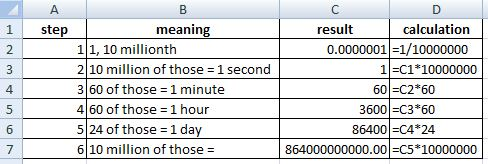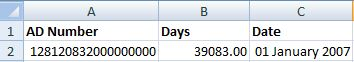# Converting Active Directory Dates to Readable Dates in Excel

This is part one of a two part article which makes use of Active Directory (AD) date and time stamps for something practical. For now though, let’s try to understand how AD stores them and how we can interpret them. Or, if you just want to skip the brain infusion and jump to the formula, scroll to the bottom!

AD records a date and time as a number which is a count of the number of 100 nano-second intervals since the 1st of January, 1601 at zero hours. Whichever way you cut that, that’s a big number, but for the less mathematical of you (myself included), how long is a nano-second exactly?

## Definitions

Nano means billionth (from Wikipedia) and can be thought of as 10 to the power of -9 or 0.000000001 seconds. So, a billion (meaning, one thousand million) of these and you have 1 second. Since (in AD) we’re talking about the number of 100 nano-seconds, we want 100 lots of a billionth. Another way of saying that is, how many 100s go into a billion? One easy way you can find that is by simply chopping off two of the zeros (for the 100). 1, 000, 000, 000 divided by 100 -> 1, 000, 000, 000 or 10, 000, 000. So, still a lot but now we can say that AD records the number of 10 millionths of a second since the date in 1601. That helps a little but what about Excel? How does that store dates and times?

## How Does Excel Store Dates?

Excel does things slightly differently in that it records the number of days (plus bits of day as a fraction) since the 1st of January, 1900. That means one is working in nano-seconds since the 17th century and the other in days since the beginning of the 20th century. Clearly, we are going to have to do some conversion!

## Concrete Example

Let’s take a concrete example: here’s what the 1st of January, 2007 looked like in AD:

Excel on the other hand considers the same date (shown as a number and using the formatting options) to be:

## Beginning to Convert From AD to Excel

So how do we get from the AD number to the Excel number? To help illustrate it, I have made a table of the calculations:Step 1 shows us what one 10 millionth looks like as a number. If we have 10 million of those, we will have the equivalent to 1 second, which is what we do when we multiply C1 by 10000000 (result being in C2, calculation in D2).

Next we multiply that by 60 and we get the number of minutes (of course, 60 seconds = 1 minute, right?).

Step 4 shows what happens when we have 60 of those, turning that number into hours and step 5 finishes by multiplying the result by 24 to get days.

Step 6 is the interesting one because it is what you get when you have 10 million lots of the number of seconds in a day. So, to recap, step 5 told us the number of seconds in a day and step 6 takes account of the fact that really we want the number of 10 millionths (10 million of the number of seconds in a day). Is that clear? I hope so! I re-read that myself a couple of times and I am still not sure I’m being lucid enough.

So, if Excel represented dates as the number of days since the 1st of January 1601, we could just take this number and (the 864 starting number – 864 billion if you don’t think I really know!) and use that in a calculation like this:

Time to go back to our (hardening) concrete example.

Where (a) was our AD date of 1st January, 2007, (b) was the number of 10 millionths in a day and (c) is how that date is represented in Excel - 39083. Erm. Hold on. What happened to 39083? Why do we have this result over 148 thousand? Surely something is wrong?

## Taking Account of the Differing Start Dates in AD and Excel

The issue is that we still have this little problem of the fact that Excel begins in 1900 and AD starts from 1601. To solve it, the trick is to say: whatever number you get from that calculation, let’s just remove the amount of time between 1900 and 1601, in days, so that we can begin counting from 1900. And how many days is that? I’d love to show you how to get Excel to do some of the heavy lifting, especially with this hidden function, but I can’t. Excel balks at dates before 1900. Take my word for it though - the number of days is 109205 (ignoring a bug in Excel which means it ought to be 109206). Back to our answer of 148288.

If we make this into a function in Excel, we are left with:I’ve simplified that 864 billion number by using powers of 10, but it means the same thing, just shorter and easier to read. Ding,ding. We’ve reached our stop and found that was a bit of a mathematical journey, but I hope it explains things in a way that makes some kind of sense. If not, let me know and I will try to do a better job!

Hi! Did you find this useful or interesting? I have an email list coming soon, but in the meantime, if you ready anything you fancy chatting about, I would love to hear from you. You can contact me here or at stephen ‘at’ logicalmoon.com